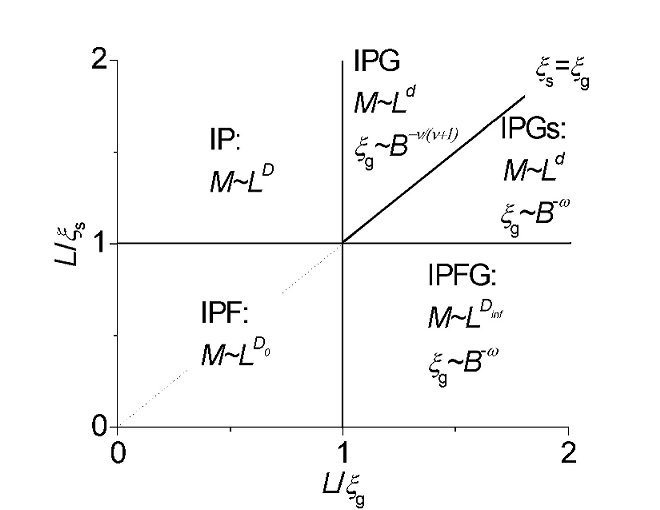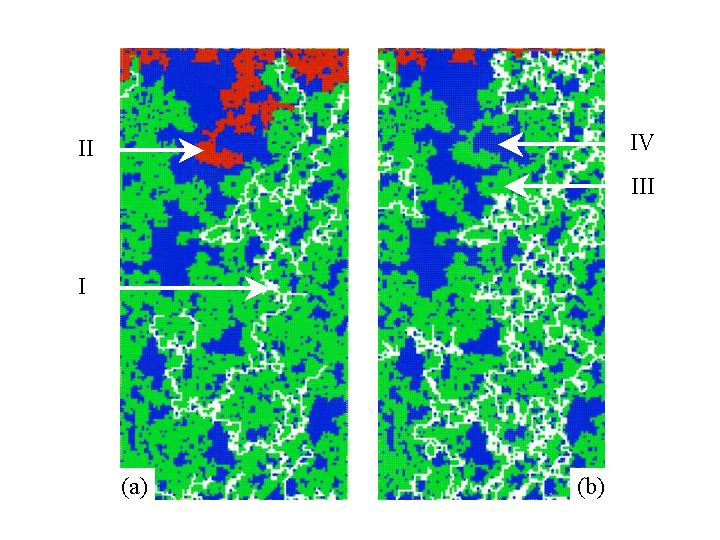Two phase flow
in fractal porous media

The influence of stabilizing hydrostatic pressure gradients on the drainage of a fractal porous medium is studied. The invasion process is treated with invasion percolation in a gradient. Fractality is mimicked by randomly closing bonds of a network. Two length scales govern the problem: the characteristic length of the pore structure xs and a length scale xg above which buoyancy determines the structure of the cluster, see figure 1.Figure 1  A schematic overview of the different scaling regimes for the mass M of the non-wetting cluster. The solid lines are the boundaries of the various regimes. In these regimes the M scales differently with the system size L. The correlation length xg scales differently with the bond number B.

When xs <xg  the local structure of the invading cluster is governed by the interplay of capillarity and the fractal properties of the pore space. Only parts of the backbone of the pore structure can be invaded. Therefore, the obtained fractal dimension for small systems L<xs is much lower, 1.40, than the one for ordinary IP, 1.82. By invasion in a fractal pore space the structure of the invader cluster is much more volatile, see figure 2.Figure 2 – The invading cluster at breakthrough in a L=100 system: a) B=0 and b) B=100. In this picture I) refers to pores filled nw-fluid, II) to pores filled with non-trapped w-fluid, III) to pores which contain trapped w-fluid and IV) to solid pores

On larger length scales, xs <L<xg, the fractality of the pore space is no longer important and the cluster grows as in ordinary IP. When L>xg, gravity becomes important and xg scales with the bond number B as xg~B^(-0.57), as in ordinary IP, while the fractal dimension becomes equal to the euclidean one.
When xg< xs gravity is already important on length scales where the fractality of the medium has to be considered too. On small scales L<xg, where only capillarity and fractality play a role the cluster structure is again characterized by the fractal dimension of 1.40. On larger length scales, xg <L< xs, gravity promotes a more efficient invasion of the pore space and different fractal dimension is found, 1.52, see figure 2. The length scale xg no longer follows ordinary IP scaling: xg ~B^(-0.69). When L>xs the fractal dimension of the invading cluster equals the euclidean one and xg ~B ^(-0.69).

• An extensive description can be found in:
• H.P. Huinink, M.A.J. Michels, The influence of buoyancy on drainage of a fractal porous medium; Physical Review E 66, 064301 (2002).